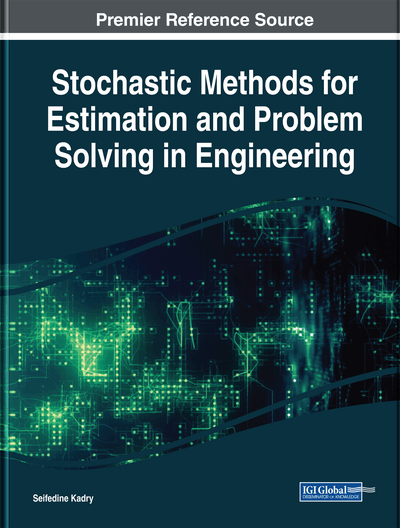# Stability of Linear Systems With Multitask Right-Hand Member

G. V. Alferov (Saint Petersburg State University, Russia), G. G. Ivanov (Saint Petersburg State University, Russia), P. A. Efimova (Saint Petersburg State University, Russia) and A. S. Sharlay (Saint Petersburg State University, Russia)
DOI: 10.4018/978-1-5225-5045-7.ch004
Available
\$37.50
No Current Special Offers

## Abstract

To study the dynamics of mechanical systems and to define the construction parameters and control laws, it is necessary to have computational models accurately describing properties of real mechanisms. From a mathematical point of view, the computational models of mechanical systems are actually the systems of differential equations. These models can contain equations that also describe non-mechanical phenomena. In this chapter, the problems of stability and asymptotic stability conditions for the motion of mechanical systems with holonomic and non-holonomic constraints are under consideration. Stability analysis for the systems of differential equations is given in term of the second Lyapunov's method. With the use of the set-theoretic approach, the necessary and sufficient conditions for stability and asymptotic stability of zero solution of the considered system are formulated. The proposed approaches can be used to study the stability of the motion for robot manipulators, transport, space, and socio-economic systems.
Chapter Preview
Top

## Introduction

Nowadays mechatronics is a field of knowledge that has a large potential. However, the unpredictability and rapid dynamics of the external environment give rise to a number of problems that are associated with the incompleteness and inconsistency of data on external world conditions. Therefore, one of the most important problems of robotic systems is a mathematical definition of the acting forces and moments in the form of motion equations. These equations are necessary to model the motion with the help of a computer and to choose the laws of motion control. In addition, they are used to assess the quality of the kinematic structure and the construction of mechanical systems. To simplify the description of the mechanical system, the components are replaced by functional converters reducing various kinds of imperfections and so on. Hence, there is an under definition in the description of mechanical systems that contain elements with incomplete information. Thus, in the study of stability of automatic control systems not only one system of differential equations is considered, but a set of systems is under consideration. These systems contain functional parameters that can be arbitrarily varied within the specified limits. In this case differential inclusions are used.

A number of interesting cases, such as the problem of absolute stability of controlled systems or the study of linear nonstationary models

where matrixelement by element satisfies inequalitycan be described by linear differential inclusions. This explains the interest in the problem of stability of linear parametrically perturbed systems. Section 1 is devoted to this problem, §§1–3.

In §1 we consider the problem of zero solution stability for a selective linear differential inclusion

whereare Hurwitz matrices of dimension.

A.F. Filippov (1985) showed that if solutionof this differential inclusion is asymptotically stable, then it is exponentially stable. A.M. Meilahs showed that asymptotic stability of the considered inclusion implies existence of a functionwhich is a Lyapunov function. He also determined an analytical form of this function. Based on the results of A.M. Meilakhs (1975), it was proved by A.P. Molchanov and E.S. Pyatnitskii (1986) that for asymptotic stability of equilibrium positionof differential inclusion it is necessary and sufficient a natural number m, a numberand symmetric matrices,…,to exist, such that function

satisfies the following relation for all xwheredenotes the derivative of the functionin the direction y.

## Complete Chapter List

Search this Book:
Reset